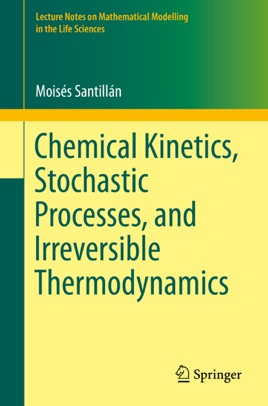• 32,99 €

## Descrizione dell’editore

This book brings theories in nonlinear dynamics, stochastic processes, irreversible thermodynamics, physical chemistry, and biochemistry together in an introductory but formal and comprehensive manner.  Coupled with examples, the theories are developed stepwise, starting with the simplest concepts and building upon them into a more general framework.  Furthermore, each new mathematical derivation is immediately applied to one or more biological systems.  The last chapters focus on applying mathematical and physical techniques to study systems such as: gene regulatory networks and ion channels.

The target audience of this book are mainly final year undergraduate and graduate students with a solid mathematical background (physicists, mathematicians, and engineers), as well as with basic notions of biochemistry and cellular biology.  This book can also be useful to students with a biological background who are interested in mathematical modeling, and have a working knowledge of calculus, differential equations, and a basic understanding of probability theory.​